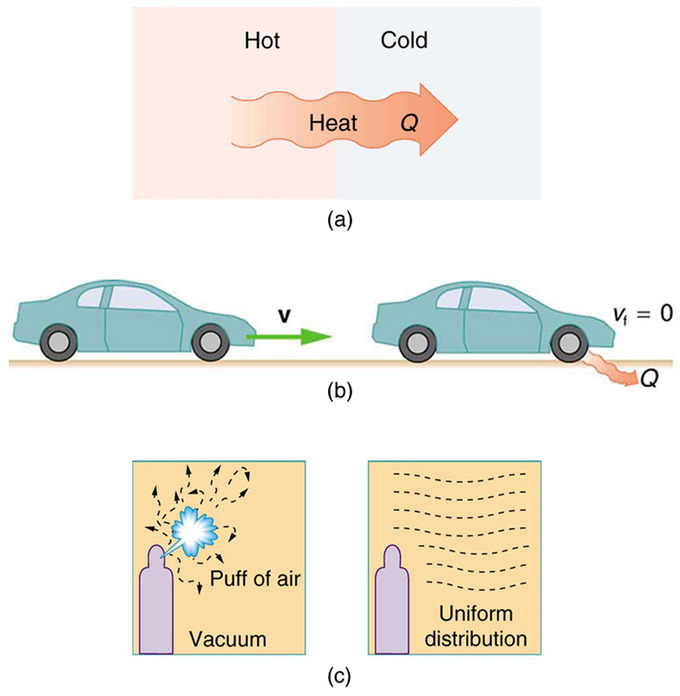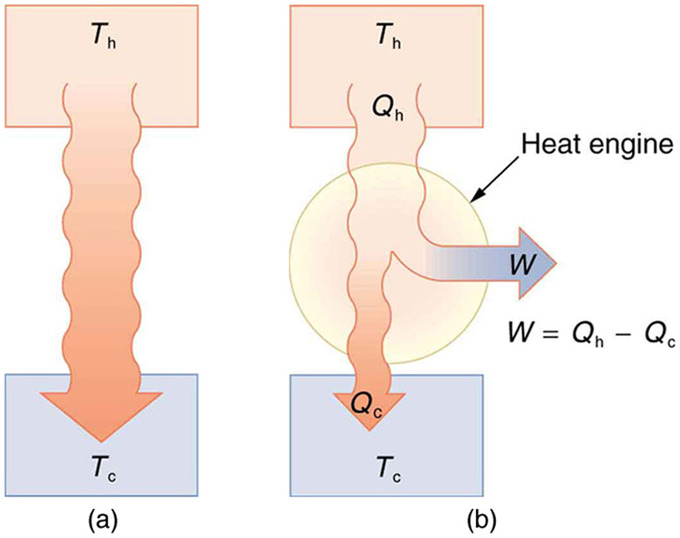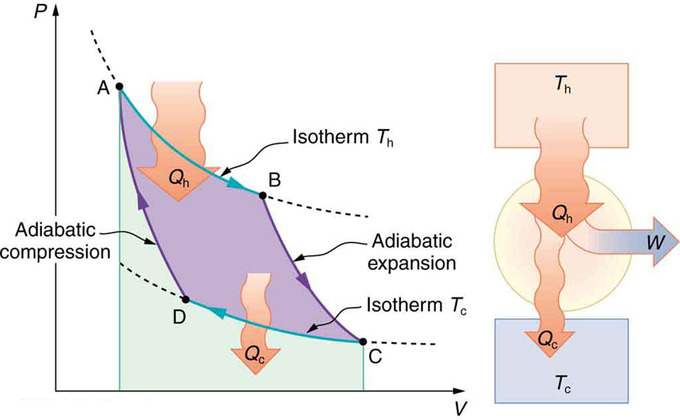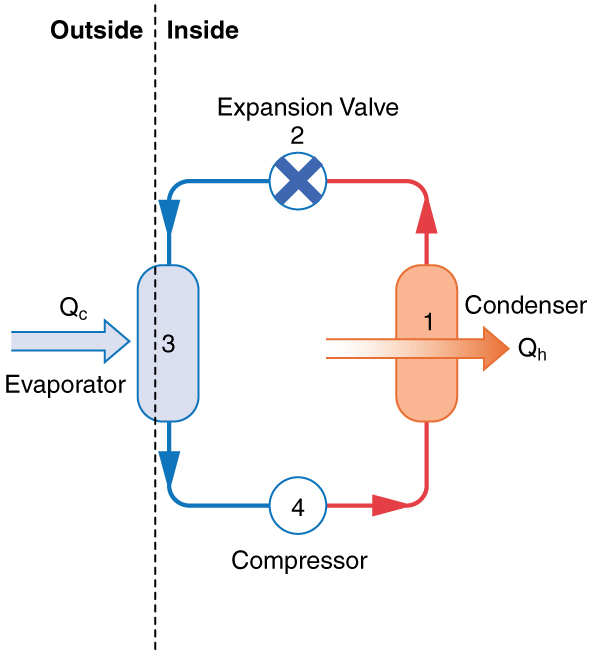The Second Law

The second law of thermodynamics states that heat transfer occurs spontaneously only from higher to lower temperature bodies.

Learning Objectives

Contrast the concept of irreversibility between the First and Second Laws of Thermodynamics

Key Takeaways

Key Points

• Many thermodynamic phenomena, allowed to occur by the first law of thermodynamics, never occur in nature.
• Many processes occur spontaneously in one direction only, and the second law of thermodynamics deals with the direction taken by spontaneous processes.
• According to the second law of thermodynamics, it is impossible for any process to have heat transfer from a cooler to a hotter object as its sole result.

Key Terms

• entropy: A measure of how evenly energy (or some analogous property) is distributed in a system.
• the first law of thermodynamics: A version of the law of conservation of energy, specialized for thermodynamical systems. Usually expressed as ΔU=Q−W.

Irreversibility

The second law of thermodynamics deals with the direction taken by spontaneous processes. Many processes occur spontaneously in one direction only—that is, they areirreversible, under a given set of conditions. Although irreversibility is seen in day-to-day life—a broken glass does not resume its original state, for instance—complete irreversibility is a statistical statement that cannot be seen during the lifetime of the universe. More precisely, an irreversible process is one that depends on path. If the process can go in only one direction, then the reverse path differs fundamentally and the process cannot be reversible.

For example, heat involves the transfer of energy from higher to lower temperature. A cold object in contact with a hot one never gets colder, transferring heat to the hot object and making it hotter. Furthermore, mechanical energy, such as kinetic energy, can be completely converted to thermal energy by friction, but the reverse is impossible. A hot stationary object never spontaneously cools off and starts moving. Yet another example is the expansion of a puff of gas introduced into one corner of a vacuum chamber. The gas expands to fill the chamber, but it never regroups in the corner. The random motion of the gas molecules could take them all back to the corner, but this is never observed to happen.One-Way Processed in Nature: Examples of one-way processes in nature. (a) Heat transfer occurs spontaneously from hot to cold and not from cold to hot. (b) The brakes of this car convert its kinetic energy to heat transfer to the environment. The reverse process is impossible. (c) The burst of gas let into this vacuum chamber quickly expands to uniformly fill every part of the chamber. The random motions of the gas molecules will never return them to the corner.

Second Law of Thermodynamics

The fact that certain processes never occur suggests that there is a law forbidding them to occur. The first law of thermodynamics would allow them to occur—none of those processes violate conservation of energy. The law that forbids these processes is called the second law of thermodynamics. We shall see that the second law can be stated in many ways that may seem different, but these many ways are, in fact, equivalent. Like all natural laws, the second law of thermodynamics gives insights into nature, and its several statements imply that it is broadly applicable, fundamentally affecting many apparently disparate processes. The already familiar direction of heat transfer from hot to cold is the basis of our first version of the second law of thermodynamics.

Thermodynamics and Heat Engines: A brief introduction to heat engines and thermodynamic concepts such as the Carnot Engine for students.

The Second Law of Thermodynamics(first expression): Heat transfer occurs spontaneously from higher- to lower-temperature bodies but never spontaneously in the reverse direction.

The law states that it is impossible for any process to have as its sole result heat transfer from a cooler to a hotter object. We will express the law in other terms later on, most importantly in terms of entropy.

Heat Engines

In thermodynamics, a heat engine is a system that performs the conversion of heat or thermal energy to mechanical work.

Learning Objectives

Justify why efficiency is one of the most important parameters for any heat engine

Key Takeaways

Key Points

• A cyclical process brings a system, such as the gas in a cylinder, back to its original state at the end of every cycle. Most heat engines, such as reciprocating piston engines and rotating turbines, use cyclical processes.
• The second law of thermodynamics can be expressed as the following: It is impossible in any system for heat transfer from a reservoir to completely convert to work in a cyclical process in which the system returns to its initial state.
• The efficiency of a heat engine (Eff) is defined to be the engine’s net work output W divided by heat transfer to the engine: $\text{Eff} = \frac{\text{W}}{\text{Q}_\text{h}} = 1 - \frac{\text{Q}_\text{c}}{\text{Q}_\text{h}}$, where Qc and Qh denotes heat transfer to hot (engine) and cold (environment) reservoir.

Key Terms

• thermal energy: The internal energy of a system in thermodynamic equilibrium due to its temperature.
• internal energy: The sum of all energy present in the system, including kinetic and potential energy; equivalently, the energy needed to create a system, excluding the energy necessary to displace its surroundings.

In thermodynamics, a heat engine is a system that performs the conversion of heat or thermal energy to mechanical work. Gasoline and diesel engines, jet engines, and steam turbines are all heat engines that do work by using part of the heat transfer from some source. Heat transfer from the hot object (or hot reservoir) is denoted as Qh, while heat transfer into the cold object (or cold reservoir) is Qc, and the work done by the engine is W. The temperatures of the hot and cold reservoirs are Th and Tc, respectively.Heat Transfer: (a) Heat transfer occurs spontaneously from a hot object to a cold one, consistent with the second law of thermodynamics. (b) A heat engine, represented here by a circle, uses part of the heat transfer to do work. The hot and cold objects are called the hot and cold reservoirs. Qh is the heat transfer out of the hot reservoir, W is the work output, and Qc is the heat transfer into the cold reservoir.

Thermodynamics and Heat Engines: A brief introduction to heat engines and thermodynamic concepts such as the Carnot Engine for students.

Because the hot reservoir is heated externally, which is energy intensive, it is important that the work is done as efficiently as possible. In fact, we would like W to equal Qh, and for there to be no heat transfer to the environment (Qc=0). Unfortunately, this is impossible. The second law of thermodynamics (second expression) also states, with regard to using heat transfer to do work: It is impossible in any system for heat transfer from a reservoir to completely convert to work in a cyclical process in which the system returns to its initial state.

A cyclical process brings a system, such as the gas in a cylinder, back to its original state at the end of every cycle. Most heat engines, such as reciprocating piston engines and rotating turbines, use cyclical processes. The second law, in its second form, clearly states that such engines cannot have perfect conversion of heat transfer into work done.

Efficiency

A cyclical process brings the system back to its original condition at the end of every cycle. By definition, such a system’s internal energy U is the same at the beginning and end of every cycle—that is, ΔU=0. The first law of thermodynamics states that ΔU=Q−W, where Q is the net heat transfer during the cycle (Q=Qh−Qc) and W is the net work done by the system. Since ΔU=0 for a complete cycle, we have W=Q. Thus the net work done by the system equals the net heat transfer into the system, or

$\text{W} = \text{Q}_\text{h} - \text{Q}_\text{c}$ (cyclical process),

just as shown schematically in (b).

Efficiency is one of the most important parameters for any heat engine. The problem is that in all processes, there is significant heat transfer Qc lost to the environment. In the conversion of energy to work, we are always faced with the problem of getting less out than we put in. We define the efficiency of a heat engine (Eff) to be its net work output W divided by heat transfer to the engine Qh:

$\text{Eff} = \frac{\text{W}}{\text{Q}_\text{h}}$.

Since W=Qh−Qc in a cyclical process, we can also express this as

$\text{Eff} = \frac{\text{Q}_\text{h} - \text{Q}_\text{c}}{\text{Q}_\text{h}} = 1 - \frac{\text{Q}_\text{c}}{\text{Q}_\text{h}}$ (for cyclical process),

making it clear that an efficiency of 1, or 100%, is possible only if there is no heat transfer to the environment (Qc=0).

Carnot Cycles

The Carnot cycle is the most efficient cyclical process possible and uses only reversible processes through its cycle.

Learning Objectives

Analyze why the Carnot engine is considered the perfect engine

Key Takeaways

Key Points

• The second law of thermodynamics indicates that a Carnot engine operating between two given temperatures has the greatest possible efficiency of any heat engine operating between these two temperatures.
• Irreversible processes involve dissipative factors, which reduces the efficiency of the engine. Obviously, reversible processes are superior from the efficiency perspective.
• Carnot efficiency, the maximum achievable heat engine efficiency, is given as $\text{Eff}_\text{c} = 1-\frac{\text{T}_\text{c}}{\text{T}_\text{h}}$.

Key Terms

• the second law of thermodynamics: A law stating that states that the entropy of an isolated system never decreases, because isolated systems spontaneously evolve toward thermodynamic equilibrium—the state of maximum entropy. Equivalently, perpetual motion machines of the second kind are impossible.
• heat engine: Any device which converts heat energy into mechanical work.

We know from the second law of thermodynamics that a heat engine cannot be 100 percent efficient, since there must always be some heat transfer Qc to the environment. (See our atom on “Heat Engines. “) How efficient can a heat engine be then? This question was answered at a theoretical level in 1824 by a young French engineer, Sadi Carnot (1796-1832), in his study of the then-emerging heat engine technology crucial to the Industrial Revolution. He devised a theoretical cycle, now called the Carnot cycle, which is the most efficient cyclical process possible. The second law of thermodynamics can be restated in terms of the Carnot cycle, and so what Carnot actually discovered was this fundamental law. Any heat engine employing the Carnot cycle is called a Carnot engine.

What is crucial to the Carnot cycle is that only reversible processes are used. Irreversible processes involve dissipative factors, such as friction and turbulence. This increases heat transfer Qc to the environment and reduces the efficiency of the engine. Obviously, then, reversible processes are superior.

The second law of thermodynamics (a third form): A Carnot engine operating between two given temperatures has the greatest possible efficiency of any heat engine operating between these two temperatures. Furthermore, all engines employing only reversible processes have this same maximum efficiency when operating between the same given temperatures.

Efficiency

The Carnot cycle comprises two isothermal and two adiabatic processes. Recall that both isothermal and adiabatic processes are, in principle, reversible.PV Diagram for a Carnot Cycle: PV diagram for a Carnot cycle, employing only reversible isothermal and adiabatic processes. Heat transfer Qh occurs into the working substance during the isothermal path AB, which takes place at constant temperature Th. Heat transfer Qc occurs out of the working substance during the isothermal path CD, which takes place at constant temperature Tc. The net work output W equals the area inside the path ABCDA. Also shown is a schematic of a Carnot engine operating between hot and cold reservoirs at temperatures Th and Tc.

Carnot also determined the efficiency of a perfect heat engine—that is, a Carnot engine. It is always true that the efficiency of a cyclical heat engine is given by: $\text{Eff} = \frac{\text{Q}_\text{h} - \text{Q}_\text{c}}{\text{Q}_\text{h}} = 1-\frac{\text{Q}_\text{c}}{\text{Q}_\text{h}}$.

What Carnot found was that for a perfect heat engine, the ratio Qc/Qh equals the ratio of the absolute temperatures of the heat reservoirs. That is, Qc/Qh=Tc/Th for a Carnot engine, so that the maximum or Carnot efficiency EffC is given by $\text{Eff}_\text{c} = 1-\frac{\text{T}_\text{c}}{\text{T}_\text{h}}$, where Th and Tc are in kelvins. (Derivation of the formula is slightly beyond the scope of this atom. ) No real heat engine can do as well as the Carnot efficiency—an actual efficiency of about 0.7 of this maximum is usually the best that can be accomplished.

Heat Pumps and Refrigerators

A heat pump is a device that transfers heat energy from a heat source to a heat sink against a temperature gradient.

Learning Objectives

Explain how the the components of a heat pump cause heat to transfer from a cold reservoir to a hot reservoir

Key Takeaways

Key Points

• A heat pump ‘s mission is for heat transfer Qh to occur into a warm environment, such as a home in the winter.
• The mission of air conditioners and refrigerators is for heat transfer Qc to occur from a cool environment, such as chilling a room or keeping food at lower temperatures than the environment.
• A heat pump can be used both to heat and cool a space. It is essentially an air conditioner and a heating unit all in one. This is made possible by reversing the flow of its refrigerant, changing the direction net heat transfer.

Key Terms

• CFC: An organic compound that was commonly used as a refrigerant. Not commonly used anymore because of its ozone depletion effect.

Heat pumps, air conditioners, and refrigerators utilize heat transfer from cold to hot. Heat transfer (Qc) occurs from a cold reservoir and into a hot one. This requires work input W, which is also converted to heat transfer. Thus the heat transfer to the hot reservoir is Qh=Qc+W. A heat pump’s mission is for heat transfer Qh to occur into a warm environment, such as a home in the winter. The mission of air conditioners and refrigerators is for heat transfer Qc to occur from a cool environment, such as chilling a room or keeping food at lower temperatures than the environment. Actually, a heat pump can be used both to heat and cool a space. It is essentially an air conditioner and a heating unit all in one. In this section we will concentrate on its heating mode.

Heat Pumps

A working fluid such as a non-CFC refrigerant is used in a basic heat pump. The basic components of a heat pump in are a condenser, an expansion valve, an evaporator and a compressor. In the outdoor coils (the evaporator), heat transfer Qc occurs to the working fluid from the cold outdoor air, turning it into a gas. The electrically driven compressor (work input W) raises the temperature and pressure of the gas and forces it into the condenser coils that are inside the heated space. Because the temperature of the gas is higher than the temperature inside the room, heat transfer to the room occurs and the gas condenses to a liquid. The liquid then flows back through a pressure-reducing valve to the outdoor evaporator coils, being cooled through expansion. (In a cooling cycle, the evaporator and condenser coils exchange roles and the flow direction of the fluid is reversed. )Simple Heat Pump: A simple heat pump has four basic components: (1) condenser, (2) expansion valve, (3) evaporator, and (4) compressor.

Coefficient of Performance

The quality of a heat pump is judged by how much heat transfer Qh occurs into the warm space compared with how much work input W is required. We define a heat pump’s coefficient of performance (COPhp) to be

$\text{COP}_{\text{hp}} = \frac{\text{Q}_\text{h}}{\text{W}}$.

Since the efficiency of a heat engine is Eff=W/Qh, we see that COPhp=1/Eff. Since the efficiency of any heat engine is less than 1, it means that COPhp is always greater than 1—that is, a heat pump always has more heat transfer Qh than work put into it. Another interesting point is that heat pumps work best when temperature differences are small. The efficiency of a perfect engine (or Carnot engine) is

$\text{Eff}_\text{C}=1\frac{\text{T}_\text{c}}{\text{T}_\text{h}}$;

thus, the smaller the temperature difference, the smaller the efficiency and the greater the COPhp.

Air Conditioners and Refrigerators

Air conditioners and refrigerators are designed to cool something down in a warm environment. As with heat pumps, work input is required for heat transfer from cold to hot. The quality of air conditioners and refrigerators is judged by how much heat transfer Qc occurs from a cold environment compared with how much work input W is required. What is considered the benefit in a heat pump is considered waste heat in a refrigerator. We thus define the coefficient of performance (COPref) of an air conditioner or refrigerator to be

$\text{COP}_{\text{ref}} = \frac{\text{Q}_\text{c}}{\text{W}}$.

Since Qh=Qc+W and COPhp=Qh/W, we derive that

$\text{COP}_{\text{ref}} = \text{COP}_{\text{hp}} -1$.

Also, from Qh>Qc, we see that an air conditioner will have a lower coefficient of performance than a heat pump.